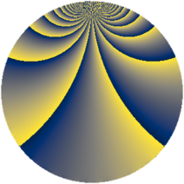# Properties

 Label 572.2.yLevel $572$ Weight $2$ Character orbit 572.y Rep. character $\chi_{572}(79,\cdot)$ Character field $\Q(\zeta_{10})$ Dimension $288$ Newform subspaces $1$ Sturm bound $168$ Trace bound $0$

# Related objects

## Defining parameters

 Level: $$N$$ $$=$$ $$572 = 2^{2} \cdot 11 \cdot 13$$ Weight: $$k$$ $$=$$ $$2$$ Character orbit: $$[\chi]$$ $$=$$ 572.y (of order $$10$$ and degree $$4$$) Character conductor: $$\operatorname{cond}(\chi)$$ $$=$$ $$44$$ Character field: $$\Q(\zeta_{10})$$ Newform subspaces: $$1$$ Sturm bound: $$168$$ Trace bound: $$0$$

## Dimensions

The following table gives the dimensions of various subspaces of $$M_{2}(572, [\chi])$$.

Total New Old
Modular forms 352 288 64
Cusp forms 320 288 32
Eisenstein series 32 0 32

## Trace form

 $$288q - 4q^{4} + 80q^{9} + O(q^{10})$$ $$288q - 4q^{4} + 80q^{9} + 8q^{12} - 6q^{14} - 32q^{16} - 50q^{18} + 16q^{22} - 88q^{25} - 50q^{28} - 20q^{30} - 4q^{33} + 30q^{36} - 48q^{37} + 38q^{38} + 70q^{40} + 56q^{42} + 66q^{44} - 96q^{45} + 70q^{46} + 76q^{48} - 56q^{49} + 70q^{50} - 20q^{52} - 80q^{53} + 44q^{56} - 20q^{57} - 2q^{58} - 144q^{60} - 150q^{62} - 100q^{64} + 30q^{66} + 24q^{69} - 28q^{70} - 100q^{72} + 40q^{73} - 140q^{74} - 24q^{77} - 40q^{78} + 118q^{80} - 76q^{81} + 56q^{82} + 120q^{84} + 80q^{85} + 44q^{88} - 8q^{89} + 80q^{90} - 14q^{92} + 72q^{93} + 50q^{94} - 140q^{96} + 116q^{97} + O(q^{100})$$

## Decomposition of $$S_{2}^{\mathrm{new}}(572, [\chi])$$ into newform subspaces

Label Dim. $$A$$ Field CM Traces $q$-expansion
$$a_2$$ $$a_3$$ $$a_5$$ $$a_7$$
572.2.y.a $$288$$ $$4.567$$ None $$0$$ $$0$$ $$0$$ $$0$$

## Decomposition of $$S_{2}^{\mathrm{old}}(572, [\chi])$$ into lower level spaces

$$S_{2}^{\mathrm{old}}(572, [\chi]) \cong$$ $$S_{2}^{\mathrm{new}}(44, [\chi])$$$$^{\oplus 2}$$